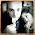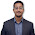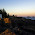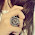### Naive Bayes Classifier in Tableau (no R/Py)

Building machine learning algorithms or predictive models in Tableau requires R or Python integration or to push the model into your ETL process. This can be difficult for some organizations who don't have this capability or want to avoid stale models. This post details how to build a Naive Bayes classification model entirely in Tableau that can scale as you feed it new data.

Naive Bayes is a probabilistic classification model based on Bayes theorem. It can be used to predict the probability of an outcome based on multiple independent conditions. It is incredibly flexible, extensible, and simple. Naive Bayes classification models can be used to detect fraud, predict attrition, or diagnose medical conditions. Really it can potentially be used to determine the probability of any event occurring

The example detailed below is a proof of concept using Titanic passenger training data from a Kaggle challenge. Below is a detailed tutorial on how to build a model in Tableau and how to apply to new data.

To build a Naive Bayes classification model in Tableau you need to create A LOT of calculated fields (nearly 30 for this example), train your model, and then blend it with a new data set in order to predict outcomes. But once this is initially setup you can implement the model in your Tableau Server environment, feed the model new data, and produce new predictions automatically. You can produce a probability for every new data point fed into your database; assuming the input variables don't change significantly.

Again the data for this example is from the Titanic Kaggle competition. The purpose of this competition is to predict whether a passenger would survive the Titanic based on their attributes (age, gender, class, etc). Competitors were provided a training set to build their model and then a test set was used to validate the accuracy.

First, you need to modify the dependent variable. In this case our dependent variable is whether or not the passenger lived in a field called Survived. This needs to be a numerical value. So I create a calculated field. You will need to do this for both outcome types: survived or died.

Next we create the prior class probability for each outcome in a field called P(Survived). The class probability is the percentage of all passengers that either survived or died. This is uses two table-scoped level of detail (LOD) expressions to calculate the total number of passengers who survived divided by all passengers. For example, 38.4% of all passengers survived the Titanic.

Then we create our series of input variables. You will need a calculated field for every input variable you want to use to train your model for both outcome types: survived and died. This variable is used to create conditional probabilities for each input. For this field we use a Fixed LOD calc based on a categorical variable as well as a table-scoped LOD expression for all passengers. The example below is for passenger class of which there are only three discrete classes: 1st, 2nd and 3rd. This field is called Class (P|Survived). This is calculating the conditional probability of a passenger surviving relative to all passengers who survived based on their class. Correction: Changed the denominator to Survived not all passengers.

I use six passenger input variables to train this model: age, class, gender, port of embarkment, # of parents/children, and # of siblings/spouses. This is 12 fields or six for each outcome type. However, after training and testing the model I only ended up using two input variables: class and gender. This produced the most accurate results.

Next, we need to calculate the prior probability of observing each condition overall regardless of outcome. This is just the percentage of all passengers who are 1st, 2nd or 3rd class and male or female. Again we use a Fixed LOD calculation for each of our input variables or Class(P) and Gender(P).

Then we need to multiply all of these probabilities together to create an overall prior probability field called (P). This is the probability of every condition combination (e.g. 1st class-female, 3rd class-male, etc).

Now we have all of the calculated fields we need to apply Bayes theorem. The following is Bayes theorem:
P(A|B) is the outcome of Bayes Theorem or our posterior probability. This is the probability of a passenger surviving given the predictors or inputs (class and gender).

P(A) is the class prior probability of a passenger surviving overall regardless of other factors. This is the probability we calculated in the first step or the field called P(Survived).

P(B|A) is the probability of a passenger being a certain class or gender given the passenger survived. This is the product of the input variables we calculated in the second step or Class (P|Survived) and Gender (P|Survived). See details below.

P(B) is the prior probability of the predictors or all the condition combinations (class and gender) of our input variables regardless of outcome. This is the field called (P) that we calculated in the last step.

To calculate P(A|B) we first need a calculated field for the numerator. This is the product of our conditional probabilities or P(B|A) and our class probability P(A). This caclulated field is called P|Survived. This again is just for survival. You need one for both survival and death.

The next steps is the final Bayes theorem: our numerator (P|Survived) divided by our probability of conditions regardless of outcome or the field (P). Again you need to do this for both outcome types.

The final probability field is to normalize the two outcomes relative to one another. Or the probability of survival versus the probability of death. This produces a clean probability out of 100%.

This is the final probability of a passenger surviving. You will need to create the same probability for death which will be the inverse or one minus this field. We can then use these fields to predict an out come. If the probability of survival is higher than the probability of death you can predict the passenger will "Survive". The reverse is true as well obviously. So we create a new field called Prediction.

In order to review and validate the model I compared the accuracy of the model prediction versus the actual results from the training set. To assess the accuracy I calculated the type 1 error. To do this I created a new field called MATCH that compares the prediction to the actual results. Accurate predictions return TRUE and inaccurate predictions FALSE. Then I count the number of passengers that were TRUE versus FALSE.

This helped me select which input variables produced the most accurate model. Ultimately, the final model only used gender and class and was 79% accurate. This means it correctly predicted a passenger's result (death or survival) 79% of the time. Compared to other model submissions from the Kaggle challenge this is low. But I could improve the model accuracy by using more variables with cleaner data but I am tired and lazy.

The following are the results of the model prediction from the training set for each passenger including the overall accuracy score.

Most importantly we want to apply this model to new data where the outcome or dependent variable is unknown. This is how the model becomes useful and scalable.

So I use the test data set from the same Kaggle challenge. I imported the new test data set into Tableau. This contains all of the same fields (except the outcome) but I only need to blend on my input variables (gender and class). I am going to link these fields and use my calculated fields from my training set created in the above steps to produce my prediction. Below is a comparison of six passengers of identical characteristics (gender and class) and how the model predicts identical outcomes for both the training and test sets.

So now I have my model blended with my new data set (test) we can produce predictions for passengers with unknown outcomes. See a detailed prediction for every passenger in my test set based on the same inputs from the training model.

In a real world environment you can feed your training model new data points as new outcomes are produced. For example, if you want to predict the probability of an employee leaving your workforce you can feed the model a training set of all employees including those who separated. Then feed your current workforce data the training model to produce a probability each current employee will leave. The model's accuracy and inputs should evolve with new data and you can adjust dynamically in Tableau as this occurs. I will write a separate post on this.

This is, in my opinion, an incredibly powerful tool to use in Tableau. Because Bayes theorem is so flexible it can be applied to a variety of business problems without altering your underlying architecture or technology stack. Your model can evolve and improve as you receive new data or stay the same depending on your business problem. Tracking the model accuracy and precision is relatively easy to do in Tableau. And because the model produces a probability, in addition to a predicted outcome, the output is relatively simple to understand for most end users.

So now anyone can build insightful predictive models in Tableau by following the above steps. But a Naive Bayes classification model will not work for every data set or business problem. So use this approach with caution and be sure to test and validate your findings.

And that's that. I would be surprised if you made it this far. This has taken me a long time to figure out so I hope this is useful. Free free to download the Tableau workbook to reverse engineer what I did or copy my calcs. If you have any questions or suggestions for how I can improve this please leave them in the comments section below. Thanks.

References:

#### 12 comments:

1.Great work Adam. Hamed's post on Visualizing the Confusion Matrix might be a good way to visualize the output of this. https://www.dataplusscience.com/VizConfusion.html

2.Thanks Jeff. That means a lot coming from you.

I like this confusion matrix viz. Always looking for better ways to visualize that.

3.> P(B|A) is the probability of a passenger being a certain age or gender given the passenger survived.

age -> class

4.Goood catch. Thank you.

5.Your work is always awesome! I learned a lot. Thanks. Just two quick questions.

1. Do you have any reasons for making a new 'Gender' term even if raw data already provides 'Sex' field?

2. According to my understanding, the term 'Class(P|Survived)' is a probability of Class 1, 2, or 3 given 'Survived' (among survived people). Thus, I think 'Class(P|Survived)' should be like this; { fixed [Pclass] : sum(Survived #) } / { sum(Survived #) }. In your calculation, denominator is { sum(Passengers) }. Do I have something wrong?

- Bryan

1.Bryan
1 - This is just a relic of the data set I used but agree it is not necessary.
2 - GREAT CATCH. Thank you. I will update.

6.This is awesome clap clap clap this is awesome :)

1.7.Posted at facebook.com/luciddataartist

8.This is Excellent!

9.Hi,

I have read your Tableau blog.It"s very attractive and impressive.Very useful to me, I like it your blog
Thanks for sharing this blog.......

Thank you
priya

10.This comment has been removed by the author.

div#ContactForm1 { display: none !important; }